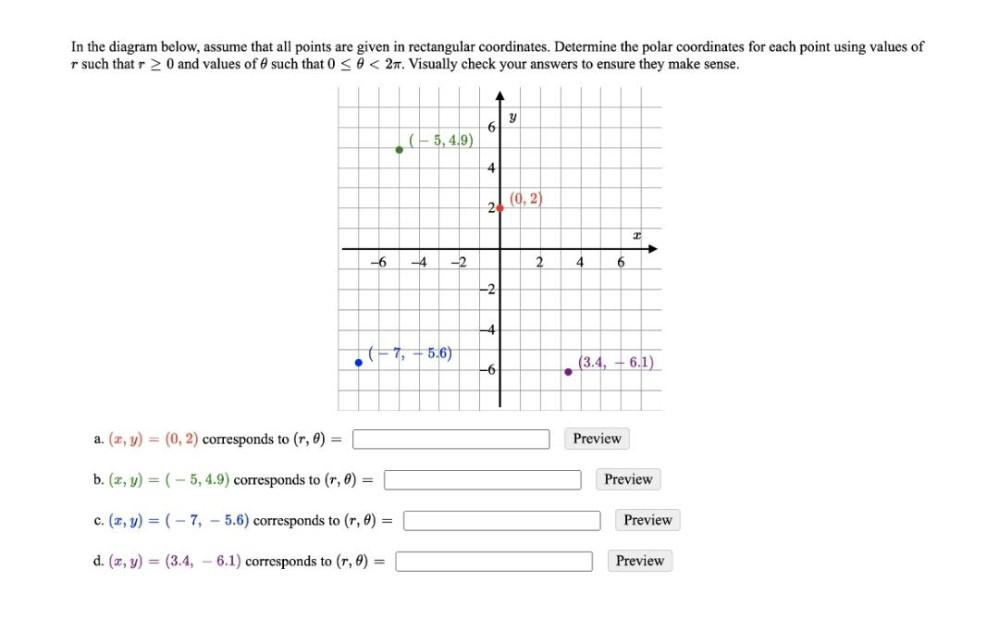Question:

# In the diagram below, assume that all points are given in rectangular coordinates. Determine the polar coordinates for each poinIn the diagram below, assume that all points are given in rectangular coordinates. Determine the polar coordinates for each point using values of r such that r> 0 and values of such that 0 <O< 27. Visually check your answers to ensure they make sense. y 6 (5,4.9) 4 2 (0,2) -6 -4 -2 2 4 6 -2 -4 7, +5.6) . (3.4, +6.1) -6 a. (2,y) = (0,2) corresponds to (r, 0) = Preview b. (x, y) = (-5,4.9) corresponds to (r, 2) = Preview c. (?, y) = (-7, - 5.6) corresponds to (r, 8) = Preview d. (2,y) = (3.4, -6.1) corresponds to (7,0) Preview